## Dividing paper into thirds, fifths, servants, and so on …

Every box-pleated origami model is based on a grid. But not all grids are made equal. Some are easier to construct than others. For instance, grids like 2×2, 4×4, 8×8, 16×16, and so on are relatively simple to make, because they could be constructed by the consecutive halving of the paper.

This is possible because their size is multiple of 2.

On the other hand, a 12×12 grid is more complex, because it can not be constructed by a consecutive halving of the paper. Nevertheless, even for this kind of grid, the procedure is simple and straightforward.

## Procedure

We need to break down the needed number of squares, in our case 12, into prime numbers, meaning, we have to split it into a group of prime numbers that, multiplied together, produce our grid size. If you have forgotten, prime numbers can be divided only by 1 and themselves. For example, prime numbers are 2, 3, 5, 7, 11, and so on.

So which prime numbers multiplied together produce 12. It is fairly easy, they are two, another two and three. So we have to divide the paper into thirds, then every third in half and then in half again. The problem is that dividing the paper into thirds is not as simple as dividing the paper in half.

What we have to do is make a crease along the diagonal. Also, we have to fold the paper along the line that connects the lower right paper corner and the middle of the left square side.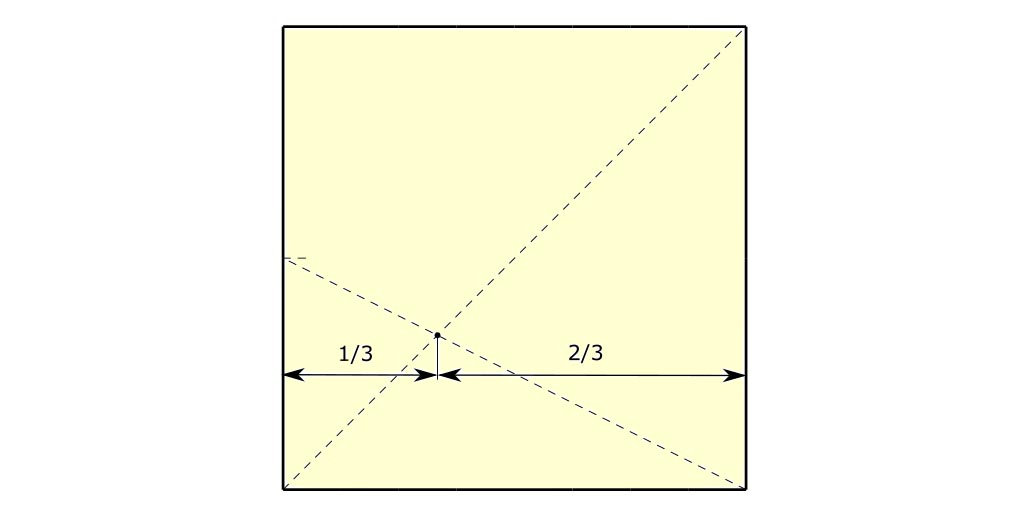Figure 1

The intersection between these two diagonal creases divides the paper into ⅓ and ⅔. Therefore, we have to make a vertical crease through the intersection. Doing that, we have to be very precise. Any misalignment will render the grid useless.

To ensure proper accuracy two additional small pinches should be made (figure 2). These two pinches will make our task much easier because making a crease through two points is way more precise.

Now that we have one crease we should make another one by dividing two-thirds into half. Doing that, we have managed to divide the paper into three thirds.

Going from paper divided into thirds to paper divided into twelfths is simple. Only what we have to do is to perform two consecutive halvings of all thirds. Nothing more.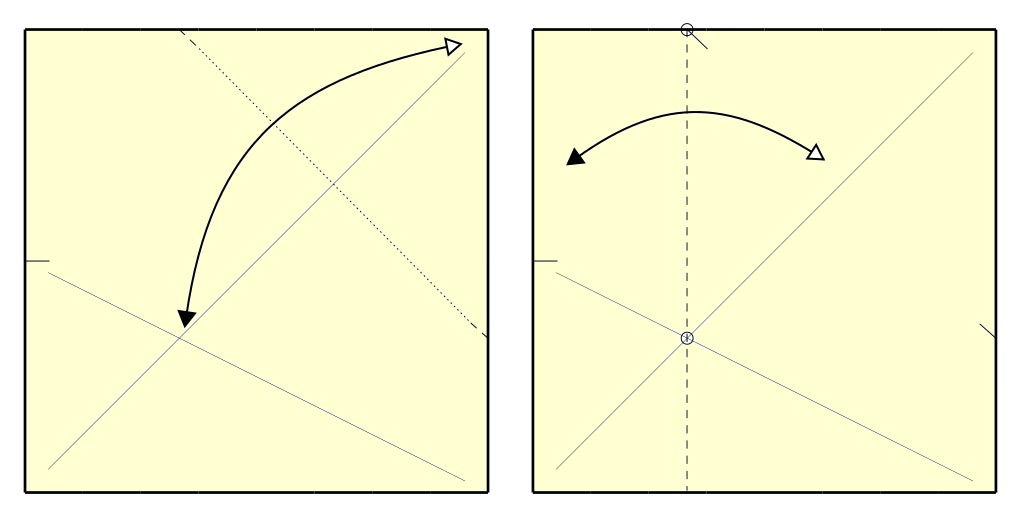Figure 2

## Geometry behind

One of the primary laws of geometry states that two triangles are considered similar if and only if all their angles are the same. I repeat: the angles are the same, not the sides or edges. If we look at our drawing, we can see that there are two such triangles. The first one has side lengths b and c (the red one).

The second one is the one having side lengths a and half of an a (the blue one).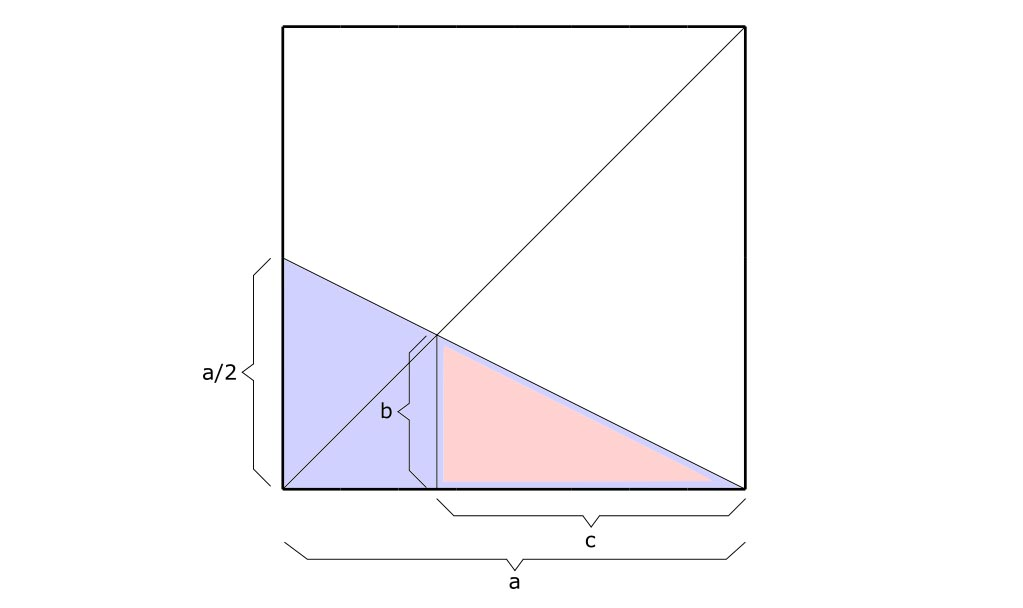Figure 3

Now, taking into consideration the fact that these triangles are similar, we know that the ratio of lengths c and b is equal to the ratio of lengths a and half of an a.

Using a simple mathematical notation this can be written like this:

c/b=a/(a/2)

… which, upon rearranging, will produce the following form:

(a-b)/b=2

An additional rearranging will lead us to this:

a-b= 2b

Finally, we’ve arrived at the result we’d been looking for: b is equal to a third of an a.

b=a/3

That is exactly what we needed. b is exactly a third of an a, which is, as you know, the length of one paper side.

## Universal procedure

I almost see you wondering, does this procedure apply to any division? The answer is strangely enough: yes.

What we need is a procedure that can make a grid of any size. I will demonstrate this so-called universal procedure by dividing the paper into sevenths. So, let’s start.

You see, regardless of the grid size, we always start by making a crease along a diagonal. That is always the first step. Now we’ve come to the important part. We need to add yet another so-called diagonal that connects the lower right corner of the paper and one point on the left edge of the paper. To find this exact point, first, we have to divide the left edge by the largest number from the group of multiples of two (2, 4, 8, 16, 32, 64, and so on) that is lower than the number of required paper partitions. That might sound complicated, but it’s not.

You see, since we want to partition the paper into sevenths, it’s obvious that we first need to divide the right edge into fourths. Seven is between 4 and 8, and since we have to choose the lower one, it is obvious that we have to choose 4?

So, our first step is to divide the right edge into fourths. Again it is enough just to make small pinches. The point we are interested in is the third one, since three is equal to the difference between seven – the number of partitions we are looking for, and four – the number of partitions we’ve actually made on the left edge. Meaning, our point is exactly three-fourths from the bottom. When we make that so-called diagonal crease, we will get an intersection that divides the paper into 3/7 and 4/7.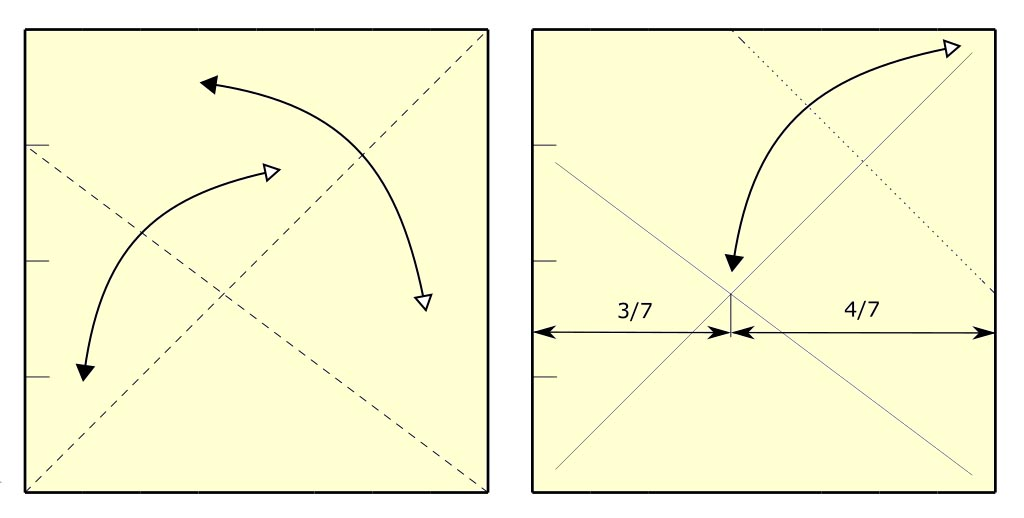Figure 4

What we have to do now is to divide 4/7 into fourths. It is quite a simple task. We already know that dividing anything into fourths is easy because four is a multiple of two.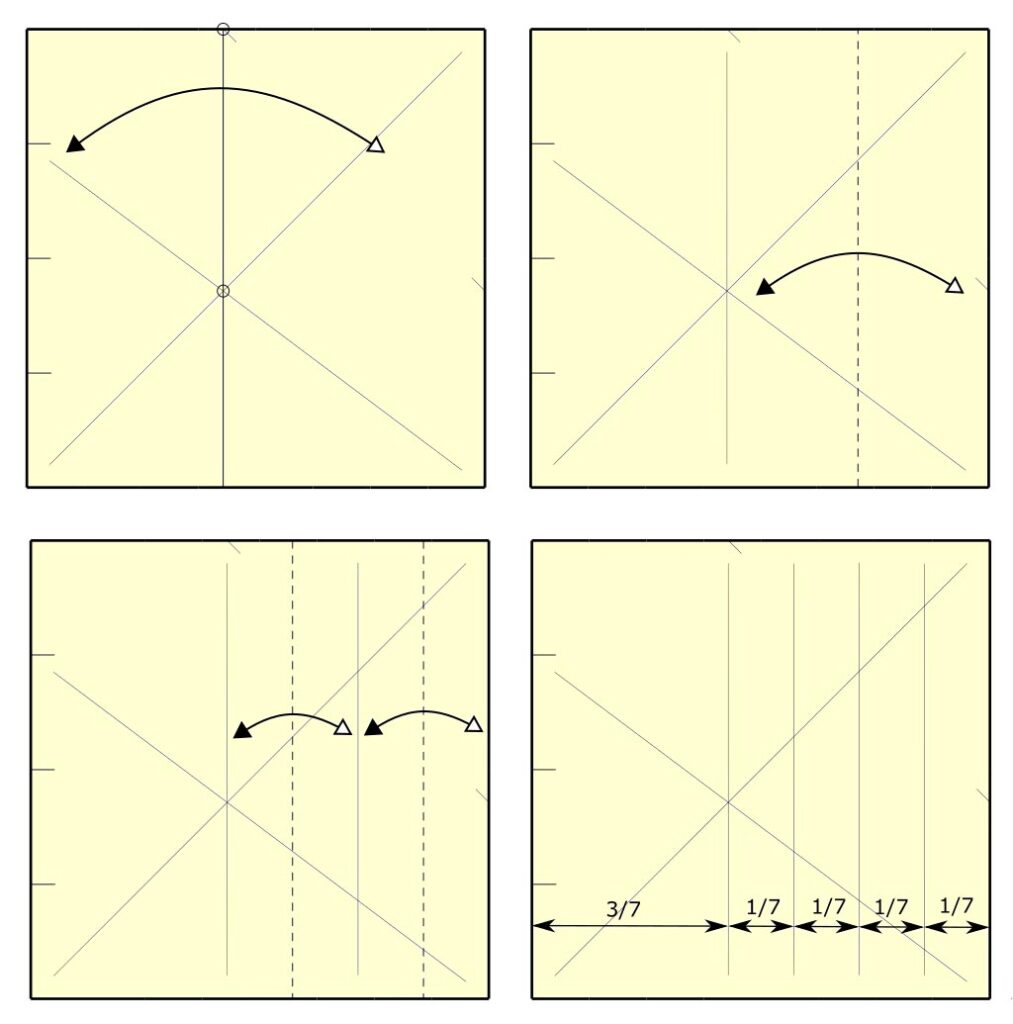Figure 5

But what about 3/7. This part of the paper should be divided into thirds. Do we have to implement the same procedure again? Of course not. What we should do is borrow one basic unit from the four-seventh segment and attach it to the three-seventh segment, thus constructing a new four-seventh segment that could be also divided by consecutive halving. As simple as that.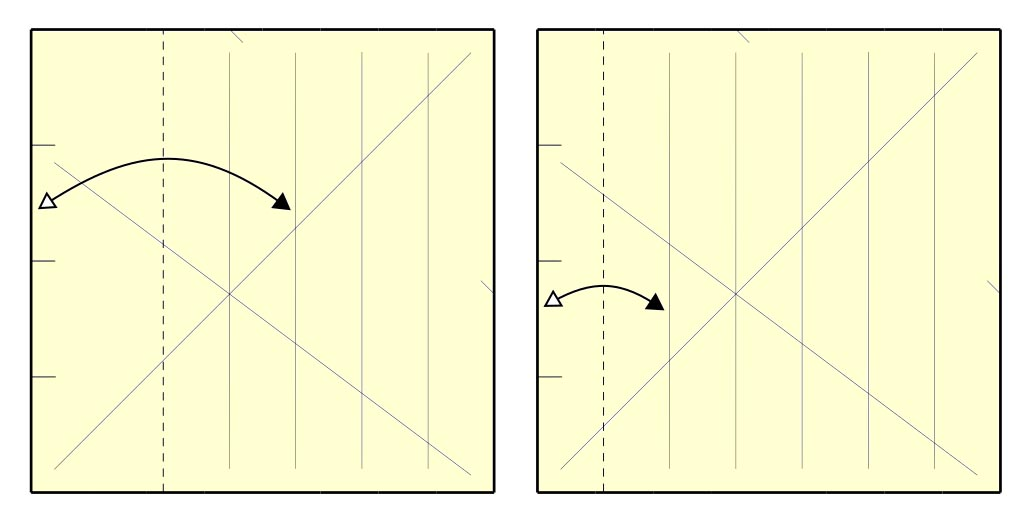Figure 6

To show you that the procedure is indeed universal, let’s create a table with all grids between 3 and 32. As you can see, the second column indicates how the left edge of the paper should be divided. The number of divisions is always the largest number from the group of multiples of two (2, 4, 8, 16) smaller than the size of the grid. For instance, for grid 13×13 it is 8 because 13 is between 8 and 16, and we have to choose the smaller one. The third column is probably the most important. It tells us, out of a certain number of marks or pinches we made on the left edge of the paper, which one will be used. In the case of the 13×13 grid is the fifth one, since the difference between thirteen (size of the grid) and eight (number of divisions we made on the left edge) is five. Finally, the last two columns tell us what kind of divisions we got. The second division is always multiple of two and, for that reason, easily divisible.

Please, pay special attention to the fact that most of the grids in the table are based on prime numbers. But there are few exceptions. For instance, a 15×15 grid is not based on a prime number (15 is not a prime number). Therefore it could be constructed as a 5×5 grid and then every partition should be further divided into thirds. Unfortunately, in this way, the complete procedure would have to be done twice. On the other hand, if we disregard the fact that 15 is not a prime number, and decide to make the 15×15 grid in one take as if it is a prime number, we would have to implement the shown procedure only once. Similar procedures can be applied to grids 9×9, 21×21, 25×25 and 27×27, even though all of them can be divided further into the smaller prime factors.

## Potential problem

From a mathematical standpoint, this method is flawless, but the implementation part in some odd cases could be a little bit tricky. The problem is that it’s absolutely essential to be very accurate if the difference between the number of partitions we are trying to make and the number of partitions we actually made on the paper edge is small. In the worst-case scenario, if it is only one.

For instance, if we want to make a 17×17 grid, we would come across such a situation. To fully understand the problem, let’s try to implement the shown procedure and try to construct a 17×17 grid. So, first, we have to divide the paper along a diagonal. That is always the first step and it is an easy one. Nex we have to construct a second diagonal. We know that 17 is between sixteen and thirty-two, so we have to divide the edge of the paper into sixteen equal sections. Now, since the difference between seventeen (grid size) and sixteen (number of partitions we made on the left edge) is one, our point of interest (pinch we made) is the first one from the bottom. I hope you see the problem. It is hard, not to say impossible, to fold a paper along this almost horizontal diagonal especially if the size of a basic unit is small.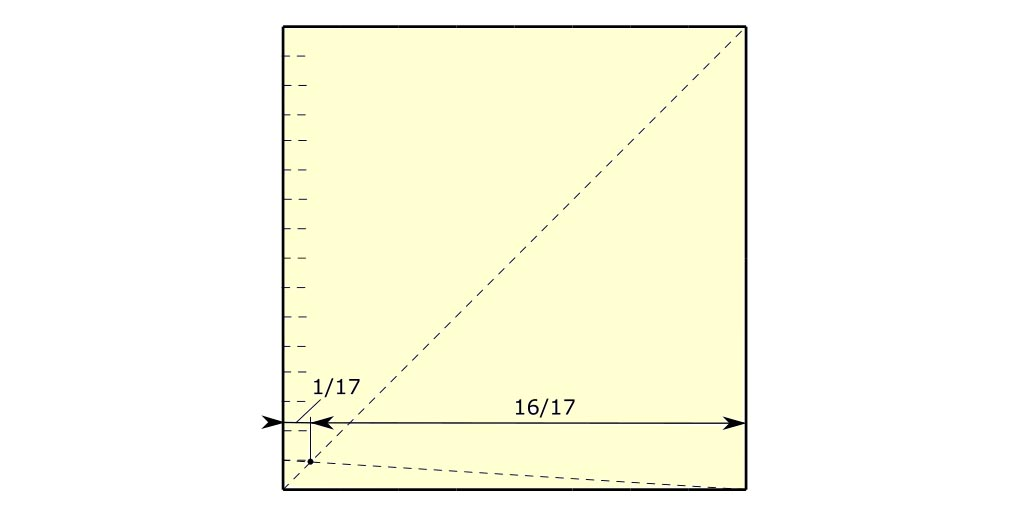Figure 7

Defining such a small basic element in a single take in such an odd configuration requires a near impossible precision. Luckily, 17×17 is only such a configuration.